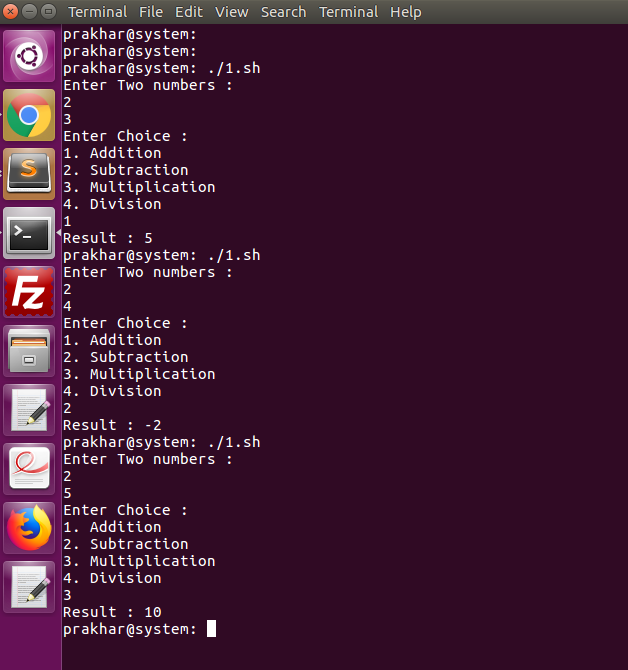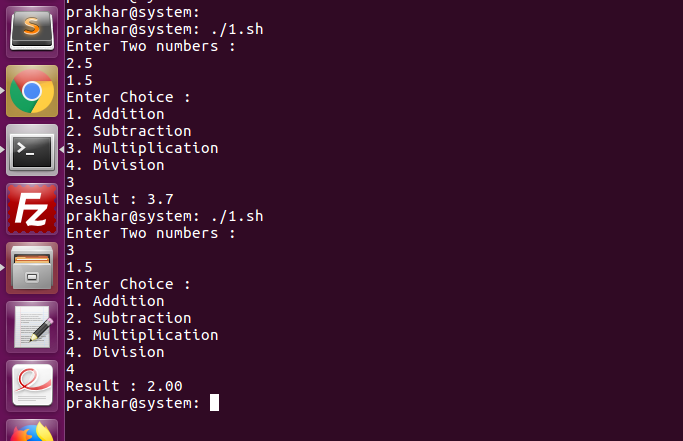# Simple Calculator in Bash

• Difficulty Level : Basic
• Last Updated : 15 Jul, 2021

Create a simple calculator which can perform basic arithmetic operations like addition, subtraction, multiplication or division depending upon the user input in Bash.

Example:

```Enter two numbers:
5.6
3.4
Enter Choice:
2. Subtraction
3. Multiplication
4. Division
3
5.6 * 3.4 = 19.0 ```

Approach:

```1. Read Two Numbers
2. Input Choice (1-Addition, 2-Subtraction, 3-Multiplication, 4-Division)
3. if Choice equals 1
Calculate res = a + b
else If Choice equals 2
Calculate res = a - b
else if Choice equals 3
Calculate res = a * b
else if Choice equals 4
Calculate res = a / b
4. Output Result, res```

Commands/Statement Used:

1. echo
echo is one of the mostly used command.
It is used to print a line of text in standard output.

`\$ echo [-neE] [arg ...] `

The command read in Linux is used to read the input from the keyboard.
3. Switch-Case
When there are a lot of if statement in Shell and it becomes confusing. Then it is good to use case statement.
4. bc Command
Checkout the link for bc Command bc Command Linux Example

## Bash

 `# !/bin/bash` `# Take user Input``echo` `"Enter Two numbers : "``read` `a``read` `b` `# Input type of operation``echo` `"Enter Choice :"``echo` `"1. Addition"``echo` `"2. Subtraction"``echo` `"3. Multiplication"``echo` `"4. Division"``read` `ch` `# Switch Case to perform``# calculator operations``case` `\$ch ``in``  ``1)res=```echo` `\$a + \$b | ``bc`````  ``;;``  ``2)res=```echo` `\$a - \$b | ``bc`````  ``;;``  ``3)res=```echo` `\$a \* \$b | ``bc`````  ``;;``  ``4)res=```echo` `"scale=2; \$a / \$b"` `| ``bc`````  ``;;``esac``echo` `"Result : \$res"`

Output:My Personal Notes arrow_drop_up